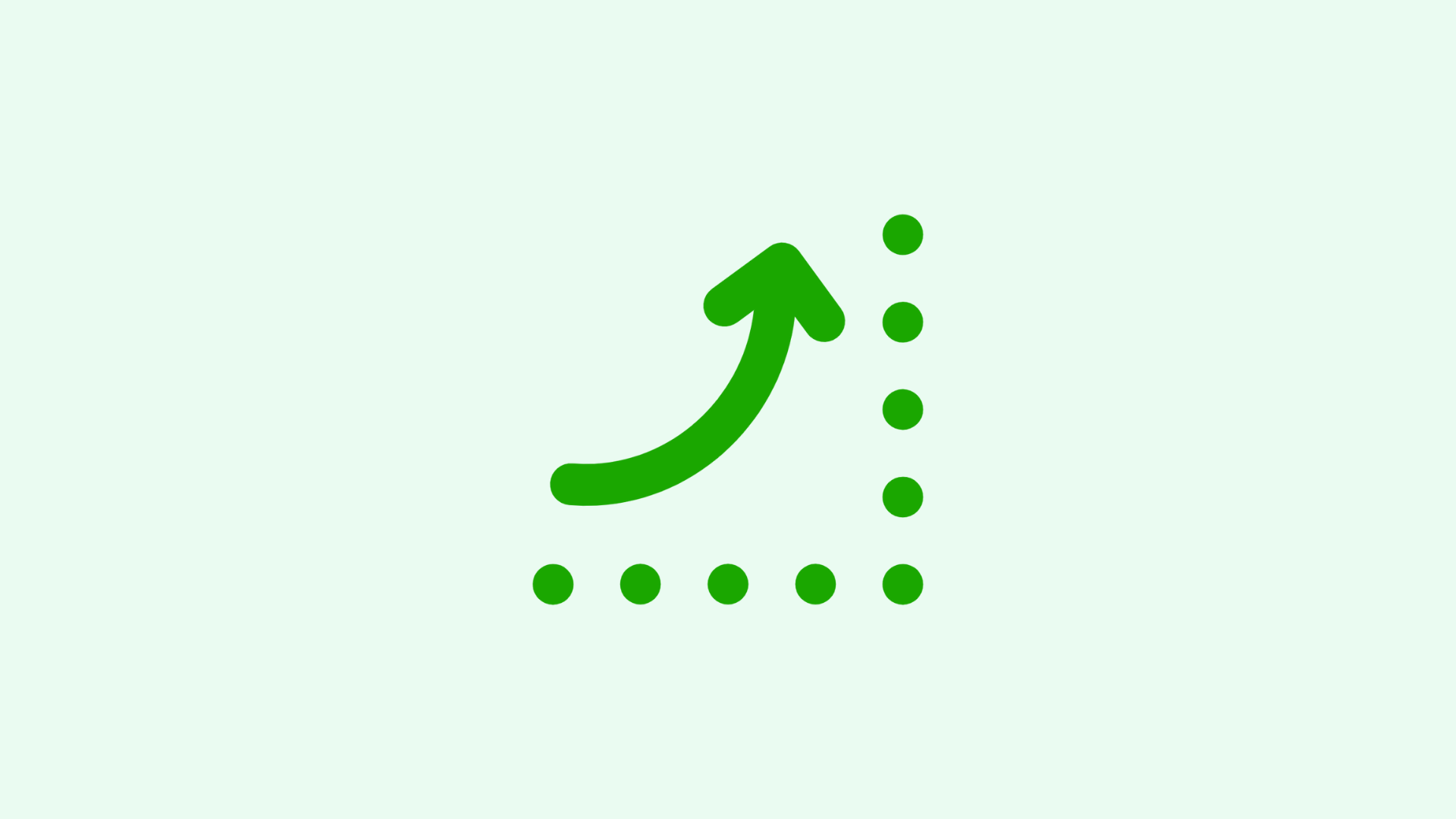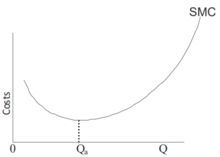# Marginal Cost: Why You Need to Know It

Timothy Ware on September 26, 2021Marginal cost is essentially the cost of producing one more unit, whether a product or service. When looking at the income statement, there are two separate types of expenses.

On the one hand, you have fixed costs. These are usually large expenses that do not change based on the number of units you produce. On the other hand, you have variable costs. These expenses are tied to the units of production and usually found under the cost of goods sold (COGS).

When dealing with unit economics, it is important to understand the differences between fixed and variable expenses and how they combine to make up your marginal cost. If you don’t know your marginal costs, then it is impossible to efficiently price your product or service.

For a SaaS business, costs are thought about differently, considering mostly the CAC (customer acquisition cost) and ACS (average cost of service), which makes things trickier, but the basic idea is the same. In this article, we go through how to calculate marginal cost, why it is important, where it is used, and then give a couple of examples of marginal cost calculations, including one using the CAC and ACS paradigm.

Baremetrics monitors subscription revenue for businesses that bring in revenue through subscription-based services. Baremetrics can integrate directly with your payment gateway, such as Stripe, and pull information about your customers and their behavior into a crystal-clear dashboard.

Baremetrics brings you metrics, dunning, engagement tools, and customer insights. Some of the things Baremetrics monitors are MRR, ARR, LTV, the total number of customers, total expenses, quick ratio, and more.

## What is marginal cost

The marginal cost is the cost to produce each additional unit of production. For example, if a company has \$10,000 in fixed costs, while the variable costs of each unit is \$10, then the marginal cost of the first unit is \$10,010, while the marginal cost of Unit 2 and onwards is \$10 until the production volume becomes so large as to put pressure on the equipment collected under fixed costs, requiring major upgrades.

This is in contrast to the unit cost, which is the average cost per unit. In this same example, the unit cost when producing one product is \$10,010, but then it drops to \$5,010 when two units are produced. Both values are important for budgeting, pricing, major capital expansion plans, etc., but be careful not to confuse them.

Furthermore, it is important to differentiate the short-run marginal cost from the long-run marginal cost. In this case, “short run” refers to a time horizon over which some costs are fixed, whereas the “long run” refers to a sufficiently long period that there are no fixed costs.

### 1. Short-run marginal cost

The short-run marginal cost refers to the basic marginal cost discussed throughout this article. It is a situation in which some costs are fixed while others are variable. For example, a company may own expensive equipment or a building which cannot be sold or replaced reasonably over a period of time. Thus, these expenses are fixed. The chart below shows the short-run marginal cost (SMC) as a U-shaped curve with the quantity on the x-axis and the cost per unit on the y-axis.

Since some costs are fixed, there is usually part of the curve on the left where the marginal cost is very high due to an inefficiently low quantity of production. Then, with economies of scale, the marginal cost of production reaches a minimum as the quantity increases.

However, without enough time to replace, upgrade, or sell fixed costs to react to an even larger volume, eventually the economies of scale reverse and the marginal cost goes up with increased production volume.### 2. Long-run marginal cost

The long run here is defined as the length of time during which no inputs are fixed. Thus, even buildings or equipment can be replaced. While the U-shaped chart above is fairly commonly seen for short-run marginal cost, there is no such predictable chart for long-run marginal cost.

It is possible that, in the long run, economies of scale continue to decrease the marginal cost, or it may remain constant or increase with scale. Furthermore, technology or management changes could have drastic positive or negative effects on the long-run marginal cost.

Baremetrics measure churn, LTV and other critical business metrics that help them retain more customers. Want to try it for yourself?

## The importance of marginal cost

The marginal cost of production is used to optimize production levels. Having a strong understanding of how costs change unit by unit gives companies the information they need to pick the production level that matches their goals.

Expanding production too quickly or too slowly can harm the bottom line. More importantly, for those companies operating in a competitive market, it tells you exactly when to stop producing more units.

## Marginal cost pricing

The marginal cost pricing strategy basically stipulates that, when operating in a competitive market, to maximize profit, a company should produce units until their short-run marginal cost is equal to their price or, vice versa, price their product at their marginal cost.

## Marginal cost formula

Marginal cost is calculated by dividing the change in costs by the change in quantity. While above an example was given of the marginal cost of the next unit, you can also think of the marginal cost of producing the next set of units, which is often more practical. Nabisco doesn’t produce one Oreo at a time, but rather an entire production run is the basic unit of increment.

Taking the example above, if the fixed costs are \$10,000 and the variables costs are \$10/unit, assuming a production decision is based on 100 units, then the marginal cost of units 101 to 202 is \$1,000/100 = \$10, whereas the marginal cost of units 1 to 100 is \$11,000/100 = \$110.

Formally, the marginal cost formula is as follows:

Marginal Cost =  (Change in Costs)/(Change in Quantity)

### 1. Change in total cost

The change in total cost is the difference between the total cost before the considered production run and the total cost after the production run. In the above example, after 100 units, the total cost is \$11,000, while it is \$12,000 after 200 units. If we are looking at the second set of units, then \$12,000 – \$11,000 leaves us at \$1,000 for the change in costs.

### 2. Change in quantity

The change in quantity is similar. It is the difference between the total quantity produced before the considered production run and the total quantity produced after the production run. In the case above, we have 100 units produced and then 200 produced. Therefore, for the second production run, the change in quantity is 200 – 100, which is 100.

## Marginal cost formula examples

While the ongoing example is pretty simple, getting all the data together to figure out the marginal cost is rarely so simple in reality. Let’s look at a couple more complicated examples to get a better idea of what all of this entails.

### 1. Basic marginal cost example

In January, you spend \$500 setting up a small online shop. It costs \$100 per month to host the shop. In the shop, you sell picture frames that cost \$3 to make and ship to the customer. In the first half of the year, you sell 1000 picture frames. By the end of July, you have sold 1300 total frames. What is the marginal cost of the frames sold in July?

First, the change in quantity is: 1300 – 1000 = 300.

Second, the cost of the frames sold from January to June is: \$500 + (\$100 × 6) + (\$3 × 1000) = \$500 + \$600 + \$3000 = \$4,100.

The total cost of the frames is: \$500 + (\$100 × 7) + (\$3 × 1300) = \$500 + \$700 + \$3,900 = \$5,100.

The change in the cost is: \$5,100 – \$4,100 = \$1000.

Third, the marginal cost is: \$1000/300 = \$3.33.

### 2. Marginal cost example in SaaS

For this example, we are going to consider a unit one month of a subscription. For your company, the CAC (customer acquisition cost) is \$500, while the ASC is \$20/month. The ASC includes everything from the amortized R&D expenses to the cost of hosting and maintaining your platform. What is the marginal cost of the first and second month?

First, the change in quantity for the first month is 1 – 0 = 1, while that of the second month is 2 – 1 = 1. That’s pretty simple.

Second, the total cost before Month 1 is \$0, before Month 2 is \$500 + \$20 = \$520, and before Month 3 is \$500 + (\$20 × 2) = \$540.

Therefore, the change in total cost for the first month is \$520 – 0 = \$520, while it is \$540 – \$520 = \$20 for the second month.

Third, the marginal cost of the first month is \$520/1 = \$520, while the marginal cost of the second month is \$20/1 = \$20.

While this summary gives you an idea of how to calculate the marginal cost in a SaaS subscription business, figuring out how to amortize your R&D over the lifetime of all your customers—not to mention matching all the other expenses to those units—is not easy!

That’s where Baremetrics comes in.

Baremetrics makes it easy to collect and visualize all of your sales data. It can be difficult to calculate your MRR, ARR, LTV, and so much more. Thankfully, there is Baremetrics to do all of this for you.